# crplot Advanced Options

## Introduction

This is the second of two vignettes on the crplot function. It details advanced features which are helpful when troubleshooting plot issues, focusing on its jump-center algorithm parameters. For a basic comprehensive summary of crplot usage, please reference the first vignette titled crplot (users should familiarize with it prior to viewing this vignette). Both vignettes are available via links on the conf package webpage.

The crplot function generates a two-dimensional confidence region plot for the specified two-parameter parametric distribution, fitted to a dataset. Details of the crplot algorithm are available in its corresponding publication1.

## Jump-Center Components

A jump-center is necessary to plot confidence region boundary points that are inaccessible via the radial profile technique (plotting algorithm of crplot) because a line joining them to the MLE crosses the confidence region boundary multiple times. The jump-center algorithm “repairs” the inaccessible region by providing an alternate point-of-reference (or alternate “center-point”, hence its name) from which to execute the radial profile plotting technique. See crplot vignette 1 of 2 for additional details.

Many advanced crplot options involve customizing its jump-center parameters. Before exploring those methods, it is first important to familiarize with jump-center components. The plot annotations below will aid in those definitions.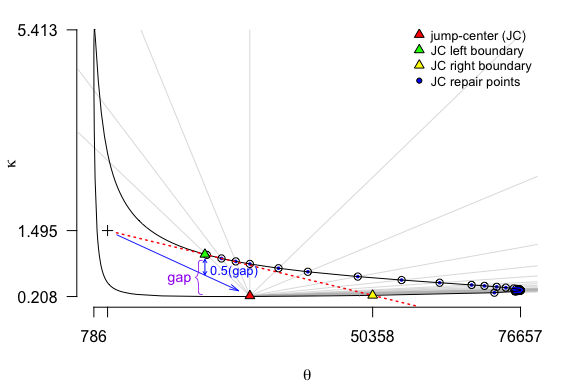(note: select word coloring below is chosen to correspond with the appropriate reference points above)

• Jump-Center & its Left and Right Boundaries. Customization is informed by the default jump-center (JC) and its JC left and JC right boundary reference points (pictured above). The “left” and “right” directional labels represent their location relative to the jump-center (when looking “out” from the jump-center into the inaccessible region). Maximum angle tolerance constraints are not satisfied at the left and/or right inaccessible region border points, and the jump-center repair aims to plot points within this uncharted region.

• Quadrant. The jump-center position with respect to the MLE ($$+$$) is significant. As a convenience, quadrant notation relative to the MLE is used. For example, the jump-center pictured above lies in the fourth quadrant relative to the MLE. All future references to quadrants within this vignette adopt this perspective (quadrants defined as if the MLE were the origin).

• Gap. Two inaccessible region orientations are possible: a vertical (shown above) or horizontal gap. The vertical gap shown above has an inaccessible region orientated counterclockwise from the MLE ($$+$$). For a jump-center in the fourth quadrant, a clockwise inaccessible region orientation corresponds to a horizontally oriented gap.

• Jump-Shift. The direction of the jump-center, relative to the MLE ($$+$$), intercepts the horizontal or vertical gap according to a jump-shift parameter. The jump-shift parameter represents the fraction of its gap where an azimuth to the jump-center crosses. The default, pictured above, is a jump-shift = 0.5 (or bisecting its gap). For this example, decreasing this value moves the azimuth from the MLE to the jump-center nearer its right boundary reference point. Increasing its value would move it nearer the left boundary.

• Jump-Uphill. The jump-center must locate inside of the existing confidence region boundary. Considering the log-likelihood function governs the landscape from which the confidence region contour is found, points inside its region are those “uphill” from its border. The jump-uphill parameter quantifies how far uphill (given as a measure of level of significance) from its confidence region boundary the jump-center locates, and thus determines its distance from the MLE (or confidence region boundary). For example, consider an $$89\%$$ versus a $$90\%$$ confidence region (or levels of significance of $$0.11$$ and $$0.1$$, respectively). The $$89\%$$ confidence region is smaller; its boundary is inside that of the $$90\%$$ region and represents a “jump-uphill” measure of $$0.01$$ (in level of significance). The default parameterization for jump-uphill is $$\min(\alpha, 0.01)$$.

## Plot errors

Plot issues using crplot are relatively uncommon, but possible. Certain datasets and/or distributions pose greater plot challenges than others. In particular, small datasets and/or heavily censored datasets can result in unusual (strong deviations from elliptical, even to the point of non-convex) shapes, making their boundary difficult to map using the radial profile log likelihood technique2 employed by crplot.

When the crplot algorithm is stretched beyond its default limitations, adjusting a series of optional arguments can further extend its practical reach. Optional arguments in the crplot function enable algorithm customization, which is often enough to overcome plot issues when they arise.

## Example

This heavily right-censored example produces a particularly challenging confidence region shape, enabling us to illustrate a suite of optional arguments available to its user to customize the crplot algorithm. Understanding the impact of optional argument adjustments will facilitate their use elsewhere when appropriate.

#### Heavily Right-Censored Dataset

Bearing cage fracture data is taken from Abernethy et. al. (1983)3. Among 1703 samples there are six observed failures. The remaining 1697 right-censored samples occur over a variety of right-censored values between 50–2050 hours. Both data subsets are given below with the variables bc_obs and bc_cen, respectively.

bc_obs <- c(230, 334, 423, 990, 1009, 1510)
bc_cen <- c(rep(50, 288), rep(150, 148), rep(250, 124), rep(350, 111), rep(450, 106),
rep(550, 99), rep(650, 110), rep(750, 114), rep(850, 119), rep(950, 127),
rep(1050, 123), rep(1150, 93), rep(1250, 47), rep(1350, 41), rep(1450, 27),
rep(1550, 11), rep(1650, 6), rep(1850, 1), rep(2050, 2))
bc <- c(bc_obs, bc_cen)
cen <- c(rep(1, length(bc_obs)), rep(0, length(bc_cen)))
print(length(bc))
#>  1703

#### Default Confidence Region Results

Confidence regions corresponding to a variety of parametric distributions for this heavily right-censored dataset are given below. Each plot uses a level of significance of alpha = 0.1 (a 90% confidence region).

distns <- c("cauchy", "gamma", "llogis", "logis", "norm", "weibull")
par(mfrow = c(2, 3))        # plot in a 2-row, 3-column grid
for (i in 1:length(distns)) {
try(crplot(dataset = bc, alpha = 0.1, distn = distns[i], cen = cen,
sf = c(0, 2), ylas = 1, main = distns[i]))
}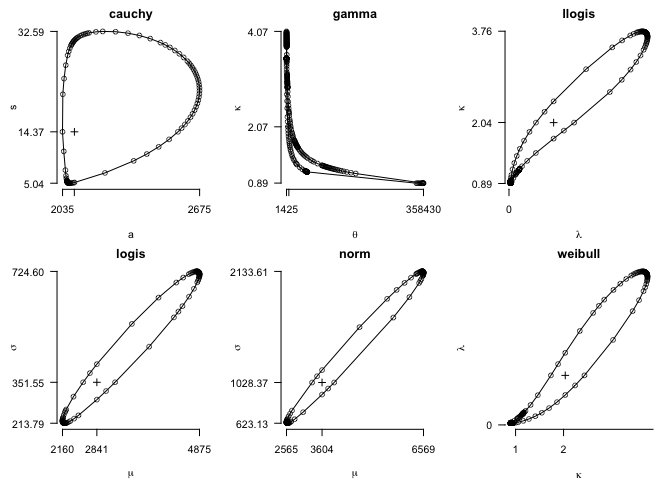#### Investigating Plot Issues

The gamma distribution confidence region plot for the bearing cage fracture dataset takes a suspect shape. Nearer the bottom of its plot there are long segments that are seemingly missing confidence region boundary points. Relatively sharp vertex angles flank each of these line segments, a characteristic indicative of a plot issue.

To assess what is happening, jump-center reference points are plot using showjump = TRUE. Additionally, its plot information is returned using the optional argument jumpinfo = TRUE. The jumpinfo argument is used in lieu of its alternative info = TRUE because we want jump-center repair information returned in addition to the final confidence region plot information. It is then available for subsequent use, as shown by plotting those points on the “without repairs” plot below.

x <- crplot(bc, alpha = 0.1, distn = "gamma", cen = cen, jumpinfo = TRUE, showjump = TRUE,
main = "with repairs")
#>  "Confidence region plot complete; made using 276 boundary points."
crplot(bc, alpha = 0.1, distn = "gamma", cen = cen, repair = FALSE,
main = "without repairs")
#>  "Confidence region plot complete; made using 134 boundary points."
jumppoints <- t(matrix(c(x$repair$q2jumpxy, x$repair$q2jumpL, x$repair$q2jumpR,
x$repair$q4jumpxy, x$repair$q4jumpL, x$repair$q4jumpR), nrow = 2))
points(jumppoints, pch = 24, bg = rep(c("red", "green", "yellow")))
print(labels(x$repair)) #>  "q2jumpuphill" "q4jumpuphill" "q2jumpshift" "q4jumpshift" #>  "q2jumpxy" "q4jumpxy" "q2jumpL" "q4jumpL" #>  "q2jumpR" "q4jumpR" "q2gaptype" "q4gaptype"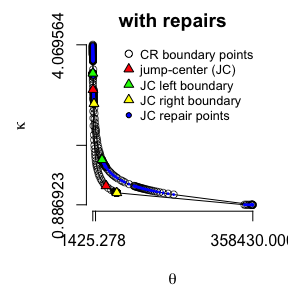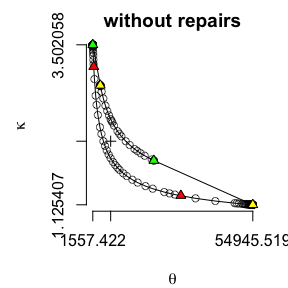Setting jumpinfo = TRUE returns a repair attribute, which is a list relevant to all jump-center repairs. The repair labels are output to the console above, and given in greater detail next. Prefixes associated with repair are: • q1, q2, q3, q4. Each label begins with an abbreviation specifying what quadrant (with respect to the MLE) the jump-center repair is made. For example, a q1 prefix indicates a jump-center repair located in the 1st quadrant (the jump-center horizontal and vertical values are both greater than the MLE’s respective values). In our example, q2 and q4 prefixes indicate jump-center repairs in the 2nd and 4th quadrant relative to the MLE (to its upper-left and lower-right, respectively). Suffixes associated with repair are: • jumpuphill quantifies the amount uphill (with regards to level of significance) from the confidence region boundary that the jump-center will locate, • jumpshift quantifies the point along the vertical or horizontal “gap” (as a fractional value between 0 and 1) that the azimuth from the MLE to the jump-center will cross, • jumpxy stores the coordinates for the jump-center, • jumpL stores the left (with perspective taken from the jump-center location towards its inaccessible region) confidence region boundary point bordering its inaccessible region, and • jumpR stores the left (with perspective taken from the jump-center location towards its inaccessible region) confidence region boundary point bordering its inaccessible region. Seeing its jump-center location enables a targeted strategy—using the optional arguments given next—to improve the plot when necessary. ### Adjusting Optional Arguments Optional arguments whose adjustment can refine crplot results include: • jumpuphill • jumpshift • ellipse_n • maxcount Optional argument adjustments can be applied separately or in combination, each serving a unique purpose. Combinations of jumpshift and/or jumpuphill are the recommended first option to address plot regions that remain inaccessible to its jump-center. The fourth quadrant repairs (with respect to the MLE) of the aforementioned example will illustrate optional argument uses below since they are more easily visible than its second quadrant repairs. #### $$\circ$$jumpuphill Increasing this optional argument from its default value, jumpuphill = min(alpha, 0.01), moves the jump-center nearer the MLE and further from its confidence region boundary. Doing so in our example will enable the fourth quadrant jump-center to achieve a better line-of-sight to the bottom portion of its confidence region (where a perceived lack of plot points appears problematic). crplot(bc, alpha = 0.1, distn = "gamma", cen = cen, jumpuphill = 0.1, showjump = TRUE) #>  "Confidence region plot complete; made using 277 boundary points."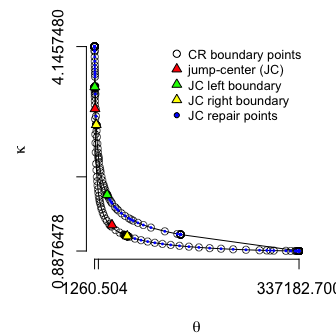The confidence region’s bottom-most curve benefits substantially from this jumpuphill adjustment, but a problematic area (lacking plot points) remains in the upper curve at higher $$\theta$$ values. #### $$\circ$$jumpshift Adjusting this optional argument from its default value, jumpshift = 0.5, shifts the radial azimuth (with respect to the MLE) to its jump-center. Decreasing its value generally “pushes” the jump-center location further along its confidence region boundary, nearer the inaccessible region. Doing so in our example enables the fourth quadrant jump-center to achieve a better line-of-sight to the upper portion of its confidence region curve at higher $$\theta$$ values (where a perceived lack of plot points appears problematic). crplot(bc, alpha = 0.1, distn = "gamma", cen = cen, jumpshift = 0.1, showjump = TRUE) #>  "Confidence region plot complete; made using 292 boundary points."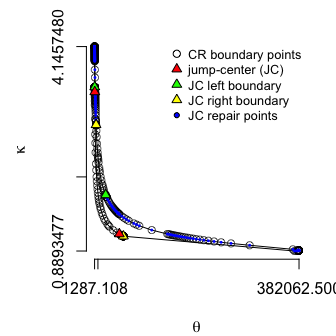The confidence region’s upper curve (at higher $$\theta$$ values) benefits substantially from this jumpshift adjustment, but does so at the expense of its bottom curve. The bottom curve (at minimal $$\kappa$$ values) plot difficulties are compounded by the perspective taken by this new jump-center location. Caution: although decreasing jumpshift generally improves access to inaccessible regions, inevitably a jumpshift value too near zero will result in multiple solutions for its jump-center at the specified jumpuphill value. Under those circumstance, the point nearer its MLE is typically returned, resulting in a jump-center whose location is not between the inaccessible boundary points, and is therefore unable to plot its inaccessible region. Using jumpshift = 0.01 rather than jumpshift = 0.1 with the syntax above demonstrates this undesirable paradigm, and is left as an exercise to the reader. Another example of a jumpshift value that is prohibitively low for its circumstance is given later in this vignette, in the Optional Argument Combinations section. #### $$\circ$$ellipse_n Adjusting this optional argument from its default value, ellipse_n = 4, combines the elliptically-oriented plot technique with the default smoothing-search algorithm. Details of the elliptically-oriented plot algorithm are given in the first crplot vignette. It increases the final number of plot points, albeit at a computational expense. Used here, it improves but does not entirely correct our example’s plot concerns. crplot(bc, alpha = 0.1, distn = "gamma", cen = cen, showjump = TRUE, ellipse_n = 80) #>  "Confidence region plot complete; made using 387 boundary points."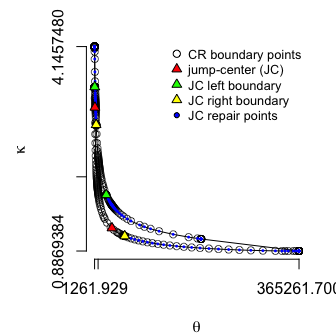#### $$\circ$$maxcount The maxcount parameter specifies the number of smoothing-search iterations crplot performs before terminating its algorithm. The default value (30) is typically sufficient to plot all accessible regions to its maximum degree tolerance constraint (maxdeg), so reaching this limit is indicative of an inaccessible region plot issue. On rare occasion however, increasing maxcount may improvement select plot regions, albeit at a noteworthy computational cost. This is true for our example plot, whose bottom region continues to (slowly) populate with relevant points as maxcount increases. crplot(bc, alpha = 0.1, distn = "gamma", cen = cen, maxcount = 50) #>  "Confidence region plot complete; made using 476 boundary points."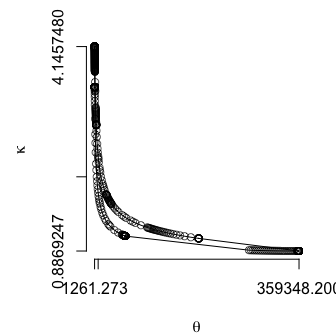Increasing maxcount is effective at identifying additional relevant points here due to poor circumstance surrounding its fourth quadrant jump-center position. The smoothing search algorithm iteratively locates additional plot points based on azimuths (or angles) associated with the mid-point of its existing points (wherever maxdeg angle constraints are not yet met). The mid-point between adjacent points along its bottom curve are so offset and spread from the jump-center that, despite aiming towards the mid-point of its long vacant region, additional points are successively added much nearer its very far-right existing point. #### $$\circ$$ Optional argument combinations Combinations of the aforementioned optional arguments are typically capable of correcting the most challenging plot circumstance. For the bearing cage fracture data example, jumpuphill and jumpshift parameter adjustments—influencing the jump-center distance and direction from the MLE, respectively—prove sufficient to adequately repair plot issues in its fourth quadrant. One solution, using jumpshift = 0.07 and jumpuphill = 0.05, is given below. x <- crplot(bc, alpha = 0.1, distn = "gamma", cen = cen, showjump = TRUE, jumpshift = 0.07, jumpuphill = 0.05, jumpinfo = TRUE) #>  "Confidence region plot complete; made using 240 boundary points." plot(c(x$theta, x$theta), c(x$kappa, x$kappa), type = "l", axes = FALSE, xlab = expression(theta), ylab = expression(kappa)) axis(side = 1, at = range(x$theta))
axis(side = 2, at = range(x$kappa))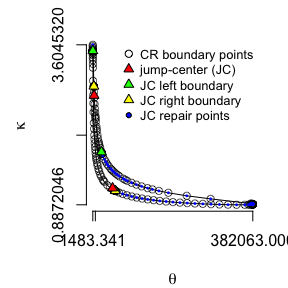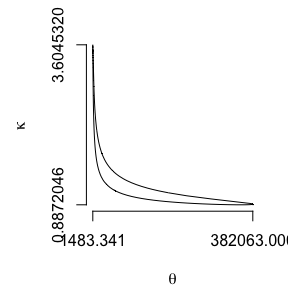A closer review of its lower-right and upper-left plot regions is appropriate to ensure those extremes are adequately captured. Those plots reveal that the quadrant four repairs were successful, but applying its customizations uniformly for all jump-center locations results in an inaccurate plot region for quadrant two. It encounters an issue discussed as “Caution: …” in the jumpshift section above. Specifically, its decreased jumpshift value causes the MLE-to-jump-center azimuth to pass too near its inaccessible region right boundary. It subsequently fails to locate its jump-center by the “far” confidence region boundary, and instead crosses another solution for the jumpuphill parameter before its inaccessible region. par(mar = c(4, 4, 1.5, 1)) # top-left confidence region extreme (zoomed-in) plot(x$theta, x$kappa, xlim = c(min(x$theta), x$thetahat), ylim = c(x$kappahat, max(x$kappa)), type = 'l', xlab = expression(theta), ylab = expression(kappa), main = "top-left (zoom-in)") # augment the top-left region graphic with plot points and jump-center information points(x$theta, x$kappa) jumppoints <- t(matrix(c(x$repair$q2jumpxy, x$repair$q2jumpL, x$repair$q2jumpR), nrow = 2)) points(jumppoints, pch = 24, bg = rep(c("red", "green", "yellow"))) # bottom-right confidence region extreme (zoomed-in) plot(x$theta, x$kappa, ylim = c(min(x$kappa), 0.95), type = 'l',
xlab = expression(theta), ylab = expression(kappa), main = "bottom-right (zoom-in)")
points(x$theta, x$kappa)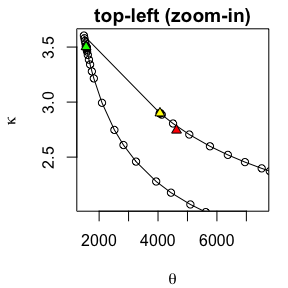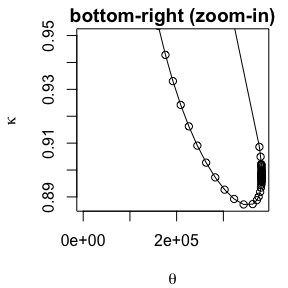Different jumpshift and jumpuphill values for quadrant two and four repairs are necessary to adequately plot each of its extreme regions to a high level of detail. Itemizing customizations in this manner is possible, and detailed in the next section.

#### Varying jumpshift and jumpuphill values by quadrant.

A plot with multiple jump-center repairs may warrant a different jumpshift and jumpuphill value for each respective jump-center. Given scalar jumpshift and/or jumpuphill arguments, the crplot algorithm assumes its value applies regardless of the jump-center repair location (or quadrant). To customize these parameters to jump-center location-specific values, assign jumpshift and/or jumpuphill a vector of length four; its values then correspond uniquely to the four respective quadrants surrounding the MLE.

When providing a quadrant-specific vector of jumpshift or jumpuphill values, any placeholder value is acceptable for quadrants with no jump-center. For our example, quadrants one and three contain no jump-center repairs and we can therefore arbitrarily assign the default values of 0.5 and 0.01 for jumpshift and jumpuphill in those respective locations.

Through separate analysis (analogous to that for quadrant four), we determine that the quadrant two jump-center adequately plots its associated region using jumpuphill = 0.25 (keeping the default value for jumpshift = 0.5). Notice how the maximum $$\kappa$$ value increases as a result. This solution is combined with the quadrant four solution (jumpshift = 0.07 and jumpuphill = 0.05) below.

# top-left confidence region extreme (zoomed-in)
x <- crplot(bc, alpha = 0.1, distn = "gamma", cen = cen, showjump  = TRUE, jumpinfo = TRUE,
jumpshift = c(0.5, 0.5, 0.5, 0.07), jumpuphill = c(0.01, 0.25, 0.01, 0.05),
xlim = c(min(x$theta), x$thetahat), ylim = c(x$kappahat, 4.15), main = "top-left (zoom-in)") #>  "Confidence region plot complete; made using 299 boundary points." # bottom-right confidence region extreme (zoomed-in) plot(x$theta, x$kappa, ylim = c(min(x$kappa), 0.95), type = 'l',
xlab = expression(theta), ylab = expression(kappa), main = "bottom-right (zoom-in)")
points(x$theta, x$kappa)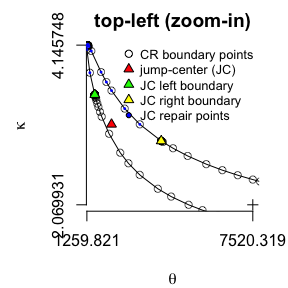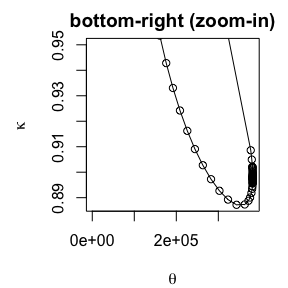Satisfied with plot accuracy in all regions, the final $$90\%%$$ confidence region for the heavily right-censored bearing fracture data is given:

# final plot, with all necessary repairs complete
plot(c(x$theta, x$theta), c(x$kappa, x$kappa), type = "l", axes = FALSE,
xlab = expression(theta), ylab = expression(kappa), main = "gamma 90% CR for BF data (final)")
axis(side = 1, at = range(x$theta)) axis(side = 2, at = range(x$kappa))
points(x$thetahat, x$kappahat, pch = 3)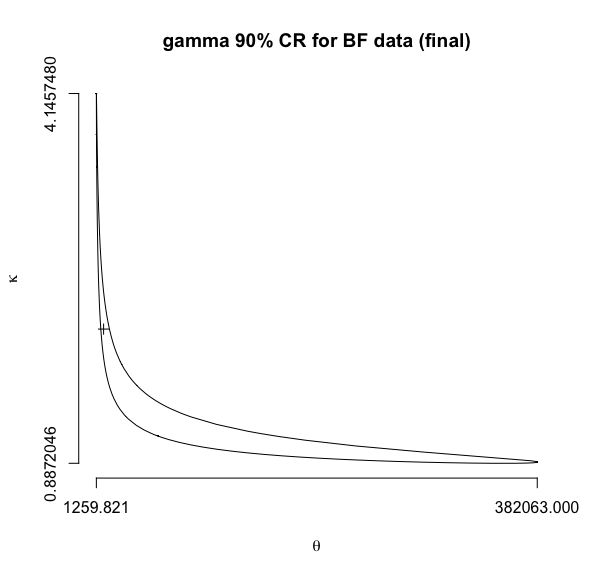## Trouble-shooting R Uniroot Errors

Albeit uncommon, if crplot is unable to return a confidence region plot the following message prints to the console:

#>  "--------------------------------------------------------------------------------------"
#>  "R uniroot failure searching for confidence region boundary---challenging parameters or shape."
#>  "Unable to produce a confidence region for the given sample and/or parameterization.   "
#>  "--------------------------------------------------------------------------------------"

The R uniroot function numerically solves for confidence region boundary points within crplot. No plot returns when this numeric method fails. Failures can occur due-to, or despite uniroot parameters upper and lower properly bracketing the confidence region (uniroot solves using a bisection method). This typically occurs under the most challenging plot circumstance such as heavily censored and/or small sample sizes, or scales that approach the limits of R’s finite precision arithmetic.

Good options to troubleshoot this error include: increasing alpha, setting repair = FALSE, and adjusting the jumpshift and/or jumpuphill parameters. Increasing alpha is effective because it reduces the confidence region size, and this is can remedy uniroot upper-bound issues. The latter two are effective options because both influence the jump-center repairs algorithm. The jump-center repairs algorithm is usually the source of uniroot errors since it addresses the most “extreme” corners of the confidence region plot, where uniroot numeric issues tend to occur. Setting repair = FALSE may enable return of a working-copy of the confidence region (complete with exception of its radially inaccessible regions). To attain a complete confidence region, adjusting jumpshift and/or jumpuphill inherently will assign its jump-center a different location, from where its uniroot calculations may not experience similar numeric difficulties.

The example below demonstrates circumstance resulting in a plot error, and attainable solutions using the strategy described above.

# crplot is unable to plot this 98% confidence region
crplot(dataset = c(1.5, 2), alpha = 0.01, distn = "invgauss")
#>  "--------------------------------------------------------------------------------------"
#>  "R uniroot failure searching for confidence region boundary---challenging parameters and/or shape."
#>  "Unable to produce a confidence region for the given sample and/or parameterization.   "
#>  "--------------------------------------------------------------------------------------"

The three troubleshooting options given above are illustrated next. For this example, each successfully produces a confidence region plot.

# a plot without jump-center repairs is attainable, but its 3rd and 4th quadrants,
# relative to the MLE, are in need of jump-center repairs
crplot(dataset = c(1.5, 2), alpha = 0.01, distn = "invgauss", repair = FALSE,
sf = c(3, 3), main = "without repairs")
#>  "99% confidence region plot complete; made using 131 boundary points."
# a complete plot is returned by increasing alpha to values >= 0.03
crplot(dataset = c(1.5, 2), alpha = 0.05, distn = "invgauss", main = "95% CR",
sf = c(3, 3))
#>  "95% confidence region plot complete; made using 276 boundary points."
# adjusting jumpshift and jumpuphill parameters
x <- crplot(dataset = c(1.5, 2), alpha = 0.02, "invgauss", jumpinfo = TRUE,
sf = c(3, 3), jumpshift = 0.1, jumpuphill = 0.001, main = "98% CR")
#>  "98% confidence region plot complete; made using 234 boundary points."
plot(c(x$mu, x$mu), c(x$lambda, x$lambda), type = "l", axes = FALSE, xlab = expression(mu),
ylab = expression(lambda), main = "98% CR")
axis(side = 1, at = format(range(x$mu), digits = 3)) axis(side = 2, at = format(range(x$lambda), digits = 3))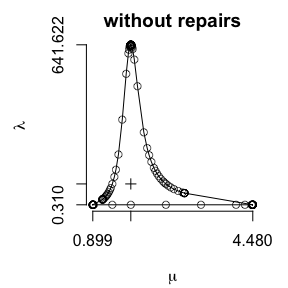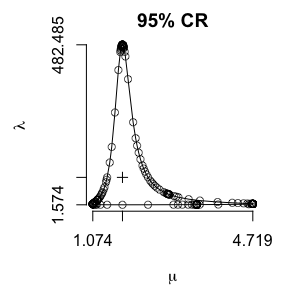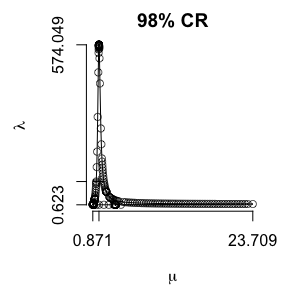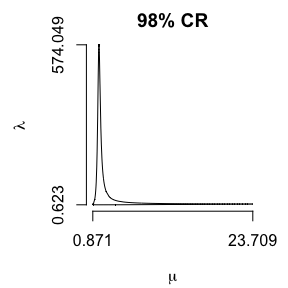1. Weld, C., Loh, A., and Leemis, L. (in press), “Plotting Likelihood-Ratio Based Confidence Regions for Two-Parameter Univariate Probability Models”, The American Statistician

2. Jaeger, A. (2016), “Computation of Two- and Three-Dimensional Confidence Regions With the Likelihood Ratio”, The American Statistician, 70, 395–398

3. Abernethy, R. B., Breneman, J. E., Medlin, C. H., and Reinman, G. L. (1983), “Weibull Analysis Handbook”, Aero Propulsion Laboratory, 43–47